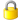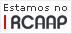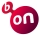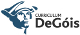Please use this identifier to cite or link to this item: http://hdl.handle.net/10174/3503

 Title: Radially increasing minimizing surfaces or deformations under pointwise constraints on positions and gradients Authors: Bicho, Luís BalsaOrnelas, António Editors: Mitidieri, Enzo Keywords: Convex calculus of variationsMultiple integralsDistributed parameter optimal controlContinuous radially symmetric monotone Issue Date: 2011 Publisher: Nonlinear Anal., Theory Methods Appl., Ser. A, Theory Methods Citation: Radially increasing minimizing surfaces or deformations under pointwise constraints on positions and gradients, Nonl. Anal. 74 (2011) 7061-7070 Abstract: In this paper, we prove existence of radially symmetric minimizers u_A(x) = U_A (|x|), having U_A(·) AC monotone and ℓ^{∗∗}(U_A(·), 0 ) increasing, for the convex scalar multiple integral \int_{B_R} ℓ^{∗∗} (u(x), |∇u(x)| ρ1 (|x|))·ρ2 (|x|) dx (∗) among those u(·) in the Sobolev space A + W_0^{1,1}. Here, |∇u(x)| is the Euclidean norm of the gradient vector and B_R is the ball {x ∈ R^d : |x| < R}; while A is the boundary data. Besides being e.g. superlinear (but no growth needed if (∗) is known to have minimum), our Lagrangian ℓ^{∗∗} : R × R → [0,∞] is just convex lsc and ∃ min ℓ^{∗∗} (R,0) and ℓ^{∗∗}(s,·) is even ∀ s; while ρ1(·) and ρ2(·) are Borel bounded away from 0 and ∞. Remarkably, (∗) may also be seen as the calculus of variations reformulation of a distributed-parameter scalar optimal control problem. Indeed, state and gradient pointwise constraints are, in a sense, built-in, since ℓ^{∗∗}(s,v) = ∞ is freely allowed. URI: http://hdl.handle.net/10174/3503 Type: article Appears in Collections: MAT - Publicações - Artigos em Revistas Internacionais Com Arbitragem Científica

Files in This Item:

File Description SizeFormat
PaperNLA1.pdf368.08 kBAdobe PDFView/OpenDSpace Software, version 1.6.2 Copyright © 2002-2008 MIT and Hewlett-Packard - Feedback